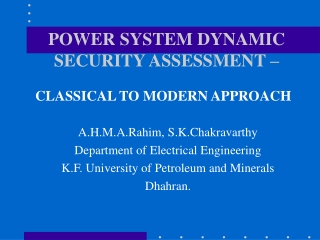DownloadDownload PresentationPOWER SYSTEM DYNAMIC SECURITY ASSESSMENT –

# POWER SYSTEM DYNAMIC SECURITY ASSESSMENT –

Télécharger la présentation## POWER SYSTEM DYNAMIC SECURITY ASSESSMENT –

- - - - - - - - - - - - - - - - - - - - - - - - - - - E N D - - - - - - - - - - - - - - - - - - - - - - - - - - -
##### Presentation Transcript

1. POWER SYSTEM DYNAMIC SECURITY ASSESSMENT – A.H.M.A.Rahim, S.K.Chakravarthy Department of Electrical Engineering K.F. University of Petroleum and Minerals Dhahran. CLASSICAL TO MODERN APPROACH

2. Background • Most power systems of today have to be operated under stressed conditions. The reserve margins available and system redundancy is gradually falling • Small scale disturbances may upset the power balance leading to isolation of a part of the system.

3. Background • Large-scale disturbances can lead to breakdown of the system. • Dynamic Security Assessment is related to addressing security related aspects pertaining to operation of a power system.

4. Dynamic Security Assessment….. • Studies the combination of outages and disturbances for ensuring stable power system operation. • The knowledge of operators are used as inputs to handle changing system toplogies, load pickup, sharp changes in weather etc. • Based on analysis of all data, contingencies are classified to assess security and take security-related decisions.

5. Dynamic Security Assessment….. • An important feature of a reliable power system is that it should supply power to the customers without causing disruption of service under all conditions. • If a severe disturbance or fault appears on the system, it should be removed quickly enough so that the system stability under the transient condition is not affected.

6. Assessing Transient Stability Index • The CCT is a complex function of the pre-fault and post fault system conditions, fault type, fault location, etc. and its determination is of paramount importance to power system planners. • Transient stability index can be assessed by numerical integration, the second method of Lyapunov, probabilistic methods, pattern recognition, expert systems or artificial neural networks.

7. The simplest way of determining the CCT is from the famous equal area criterion. Figure shows the power angle curve. The critical clearing time tcr corresponds to angle cr when area A1 equals A2. The Equal-Area Method

8. Numerical Integration • The most widely used method of determining CCT. • The system dynamics is solved repetitively until the fault duration is obtained which takes the system to the threshold of instability. • For accurate results the step size should small, making the process very slow.

9. In the second method of Lyapunov the post-fault system equations are replaced by a stability criterion. The figure shows the stable and unstable manifolds in the  -  phase plane. Lyapunov Method

10. Probabilistic Method • Generally, the determination of CCT through these methods is quite involved. • The probabilistic methods attempt to assess the probability of stability of a system following exposure to a disturbance. • In some studies, probability functions, called the security function, are compared with a maximum tolerable insecurity or risk level to determine if and when some control action has to be taken.

11. Some Modern Methods • Pattern recognition based transient stability studies, attention have been focused on selection of the initial system description, feature extraction and classifier design. • The expert system approach combines the time domain numerical approach with human expert knowledge coded in a rule based program. • The methods used in artificial neural network employ adaptive pattern recognition approach, which trains the neurons to learn from the sets of input-output presented to it.

12. For the network shown faults were simulated on buses 2 and 3. Fault-clearing policy is to restore pre-fault topology. Simulations were also made with line 2-5 being removed. Twenty different loading conditions were considered G 6 1 G 2 5 3 4 G G Application of ANN in determining CCT

13. In the training process, the network is presented with a pair of patterns – an input pattern and a corresponding desired output pattern. In the forward pass, the outputs are computed on the basis of selected weights and the error is computed. In the backward pass the weights are updated so as to minimize the sum of the squares of errors, b1 [wrm] [wpr] v1 y1 x1 ym xp vr bp Back-propagation type ANN

14. In training the RBF network the linear weights are estimated so as to minimize the sum of the square of the error between the desired output d(k) and the network output y(k). An orthogonal least squares approach chooses the centers of the radial basis functions as subsets of the weighting matrix from a linear regression model of the error equation. This method has been employed in this study. c1 wkj y1 x1 ym xp Radial Basis Type ANN

15. The rotor angle of each machine relative to the center of inertia d0 at the instant of fault initiation Accelerating power of each generator immediately following the fault Accelerating kinetic energy of each machine given as xi = di - d0 , i=1,2,…4 xi+4 = Pmi - Pei , i=1,2,…4 xi+8 = (Pmi -Pei)/ Hi , i=1,2,….4 Training data

16. Results: Convergence Characteristics

17. Results: Convergence Characteristics

18. Results: Comparison

19. Comparison of CCT

20. Conclusions • A relatively modern method of estimating CCT through artificial neural networks has been presented. • Both back-propagation as well as the radial-basis function ANN’s were trained to estimate the critical fault clearing times of a multi-machine power system. • It was observed that the RBF networks estimates the CCT with better accurately.

21. Conclusions THANK YOU FOR YOUR ATTENTION